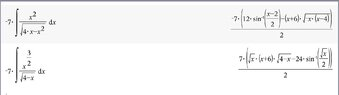# Trouble with trig integral

• MHB
• Kristal1

#### Kristal1

I have a few questions and a request for an explanation.

I worked this problem for a quite a while last night. I posted it here.

https://math.stackexchange.com/questions/3547225/help-with-trig-sub-integral/3547229#3547229

The original problem is in the top left. Sorry that the negative sometimes gets cut off in the photo, and yes I know it's not fully simplified there.

My first question is the more involved one: Is the algebra in my original work sound? If it is, why doesn't it work in this instance?

My second question is: is this a correct solution? https://imgur.com/2tgEz0O

It is for webwork, and I used two out of three chances. I'd prefer to keep my perfect webwork mark, obviously ;p

Finally, I was kind of impressed with Ans4's square completion and had to run it through to see that it was correct. That's such a useful skill. Do you have some advice about how I could improve my math tricks to that point?

Is the algebra in my original work sound?

Looks like you did this (correct me if I'm mistaken) ...

$$\displaystyle -7\int \dfrac{x^2}{\sqrt{4x-x^2}} \,dx = -7\int \dfrac{x^2}{\sqrt{x}\sqrt{4-x}} \,dx = -7\int \dfrac{x^{3/2}}{\sqrt{4-x}} \,dx$$

from here, I see you attempted to use the trig sub $\sqrt{x} = 2\sin{\theta}$.

you made a mistake determining $dx$ ...

$\sqrt{x} = 2\sin{\theta} \implies x = 4\sin^2{\theta} \implies dx = 8\sin{\theta}\cos{\theta} \, d\theta$

I did sub it in as a replacement for $\sqrt{x}$ though.

I posted this elsewhere since I wasn't even sure if it would be approved here. There it was suggested that I should have put $4cos\theta=\frac{1}{\sqrt{x}}dx$

This is an important conceptual point, though. Are you saying that this is the only correct derivative because dx means wrt x and not x to some power?

If so, well it would be nice if a teacher told us these things sometimes...

I did sub it in as a replacement for $\sqrt{x}$ though.

I posted this elsewhere since I wasn't even sure if it would be approved here. There it was suggested that I should have put $4cos\theta=\frac{1}{\sqrt{x}}dx$

This is an important conceptual point, though. Are you saying that this is the only correct derivative because dx means wrt x and not x to some power?

If so, well it would be nice if a teacher told us these things sometimes...

Hi Kristal, welcome to MHB! ;)

There are 2 ways that are generally taught to do a substitution.

You have the integral
$$\int \frac{x^{3/2}}{\sqrt{4-x}}\,dx$$
and you want to replace $\sqrt x$ by $2\sin\theta$.

Method 1
Write $x$ as a function of $\theta$ and take the derivative as skeeter suggested:
$$\sqrt x=2\sin\theta \implies x=4\sin^2\theta \implies dx=d(4\sin^2\theta) = 8\sin\theta\cos\theta\,d\theta$$
We can now replace $\sqrt x$ and $dx$ to find:
$$\int \frac{x^{3/2}}{\sqrt{4-x}}\,dx = \int \frac{(2\sin\theta)^{3}}{\sqrt{4-(2\sin\theta)^2}}\,d(4\sin^2\theta) = \int \frac{(2\sin\theta)^{3}}{\sqrt{4-(2\sin\theta)^2}}\cdot 8\sin\theta\cos\theta\,d\theta$$

Method 2
Take the derivative left and right, and do what needs to be done to eliminate $x$.
In this case:
$$\sqrt x=2\sin\theta \implies d(\sqrt x)=d(2\sin\theta) \implies \frac 1{2\sqrt x}\,dx= 2\cos\theta\,d\theta \implies \frac{dx}{\sqrt x} = 4\cos\theta\,d\theta$$
Rewrite the integral a bit to match and substitute:
$$\int \frac{x^{3/2}}{\sqrt{4-x}}\,dx = \int \frac{x^2}{\sqrt{4-x}}\cdot\frac{dx}{\sqrt x} = \int \frac{(2\sin\theta)^4}{\sqrt{4-(2\sin\theta)^2}}\cdot 4\cos\theta\,d\theta$$

We can see that the result is the same in both cases.

I'm afraid I can't comment on what your teacher is telling you since I don't know what that is.
We can only point out and show what the correct math is.

my calculator’s CAS worked out these antiderivatives ...

#### Attachments

•50048641-AAC5-4E4C-8850-48E9C774DBFC.jpeg
36.4 KB · Views: 34